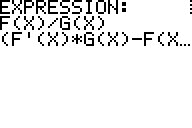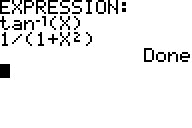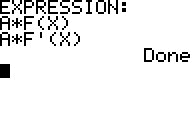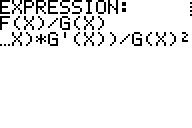Basics Archives Community Services ProgrammingHome :: Archives :: File Archives :: DerivativesDerivatives

FILE INFORMATION

derivata1.zip
 Filename derivata1.zip (Download) Title Derivatives Description ADERIV:This program gives you most sorts of derivatives, when you input their primitive functions. Use A for constant, and F(X) and G(X) for functions other than, sin, cos, tan, ln, log, e^x, 10^(x), etc. If you input F(X)/G(X), the program will give: (F'(X)G(X)-F(X)G'(X))/G(X)^2. If you input: A^X, the program gives, ln(A)*A^X. If you input: sin(X) ( Don't forget the right hand bracket! ), the program will give, cos(X). The program also has L' Hopital's rule: Input F(A)=G(A)=0, you'll see. ADERIV1: This program is a bit more advanced than ADERIV. It has a subroutine, ADERSUB that you also need to install. It is an attempt to give you support by chopping up your expression bit by bit, giving you the formulas involved. It is also more forgiving than ADERIV. Just remember not to use implicit multiplication where you want the program to give you a formula: if you type sin(x)cos(x) the program will just give you, sin(x)'=cos(x) and cos(x)'=-sin(x), but not the multiplication formula, (F(X)*G(X))'= F'(X)*G(X)+F(X)G'(X). You have to type, sin(x)*cos(x). Author Anders Tiberg (anders.tiberg@telia.com) Category TI-83/84 Plus BASIC Math Programs (Calculus) File Size 7,468 bytes File Date and Time Wed Jul 24 16:53:50 2019 Documentation Included? Yes

SCREEN SHOTSREVIEWS

There are no reviews for this file.

LEAVE FEEDBACK

Questions, comments, and problems regarding the file itself should be sent directly to the author(s) listed above.

Report inappropriate or miscategorized file (requires an account; or you may email us directly)

 Bad Good 1  2  3  4  5  6  7  8  9  10

ARCHIVE CONTENTS

Archive Contents
Name Size